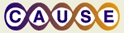# Influence of Outliers on CorrelationThis material was originally developed through CAUSE
as part of its collaboration with the SERC Pedagogic Service.

#### Summary

This activity begins with an instructor demonstration followed by a student out-of-class assignment. Students will observe their instructor create a scatterplot and observe how the correlation coefficient changes when outlier points are added. Students are then given a follow up assignment, which guides them through the applet. In addition, the assignment provides insight about outliers and their effect on correlation. This activity will show exactly how outliers numerically change the correlation coefficient value and to what degree.

## Learning Goals

• Identify points that would be considered outliers on a scatterplot.
• Given a scatterplot with an outlier marked, determine if the correlation will increase, decrease or leave the correlation unchanged.
• Students should be able to construct a scatterplot with low correlation coefficient and then add an outlier that will increase it.
• Students should be able to construct a scatterplot with high correlation coefficient and then add an outlier that will decrease it.

## Context for Use

This visualization activity is used for an introductory college statistics course. Students will be asked to complete an assignment out of class that will help them gain intuition about the influence of outliers and how they affect correlation.

Prerequisites for this activity:
• Students would have been taught about the correlation coefficient and seen several examples that match the correlation coefficient with the scatterplot.
• Students will have discussed outliers in a one variable setting.

## Description and Teaching Materials

This activity is intended to be assigned for out of class use. It can be part of a regular homework assignment or as a laboratory assignment. In this assignment students use the applet to construct specific correlations. They then move points to examine their influence on the correlation. Students summarize the main point of the exercise by answering a multiple choice question. Then the students examine a question about real data that illustrates the concept.

## Teaching Notes and Tips

Students should be encouraged to use this applet on their own to gain hands on knowledge on how correlation changes as outliers are moved around and/or removed. A step-by-step process for the students to follow is included in the follow up activity provided.

Use the applet is fairly straightforward and outlined here, or can be read on the webpage.
• When the "Add Data" button is selected, click anywhere on the plot area to add a point to the scatterplot. A point may be dragged anywhere on the plot area just by clicking and holding the left mouse button then moving the mouse. To erase a single point just drag the desired point to the trash icon.
• When enough points are placed note the correlation value in upper right portion of the applet. Also you may include the least squares regression line by clicking the "Show Least Squares Line" box. You may also show the x and y means by clicking the "Show mean X and mean Y" box.
• When "Draw line" button is selected, click-drag-release on the plot to draw a line. Drag the end-points of the line to change its slope and drag the center point of the line to move it up or down.
• The blue/green bar above the plot area gives the sum of squares for your drawn line. The smallest sums of squares possible is represent by the blue portion of the bar, so if your line matches that of the least squares regression line all that will be seen is blue, otherwise a green portion will appear representing an increased sums of squares for your drawn line.
• Finally if you wish to start over with new points, simply double click the trash icon.

## Assessment

Students can be assessed with a question on a future quiz which asks, "Which of two correlation coefficients will change the most by removing their respective outliers." Then during an upcoming regression lecture, an example of a regression will be done that has an outlier. During this discussion, the class will take a vote as to how the correlation will change as well as the regression line equation.